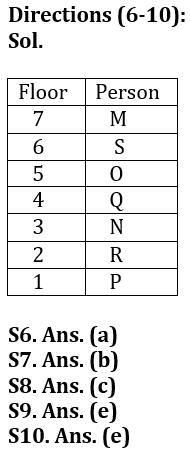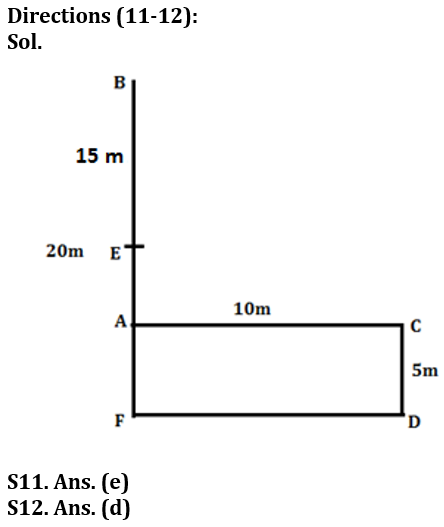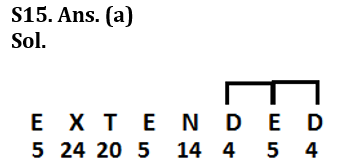Latest Banking jobs   »

# Reasoning Ability Quiz For LIC ADO Prelims 2023-2nd March

Directions (1-5): In these questions, relationships between different elements are shown in the statements. These statements are followed by two conclusions.
(a) If only Conclusion I follow
(b) If only Conclusion II follows
(c) If either Conclusion I or II follows
(d) If neither Conclusion I nor II follows
(e) If both Conclusions I and II follow

Q1. Statements: A ≥ D ≥ G ≥ H > E
Conclusions: I. A > H II. E < D

Q2. Statements: D ≥ E ≥ L , G> E ≤ F
Conclusions: I. F≥ L II. F ≥ D

Q3. Statements: U ≤ M ≤ S < R, B ≥ L = R ≤ T < D
Conclusions: I. M < D II. B > U

Q4. Statements: A > B ≥ F< C = D
Conclusions: I. D < A II. A ≥ D

Q5. Statements: K ≥ L ≥ M = N, P ≥ O ≥ N
Conclusions: I. K > P II. P ≥ K

Directions (6-10): Study the following information carefully and answer the questions given below:
Seven persons i.e., M, N, O, P, Q, R and S who all live in a seven-storey building such that ground floor is numbered 1 and above it is numbered 2 and so on till the top most floor which is numbered 7. Not more than three person lives above O. Only two person lives between M and Q. Only two person lives between R and O. N lives immediately above R. More than two person lives between N and M. S live above P, but does not live on odd numbered floor.

Q6. How many persons live above M?
(a) None
(b) One
(c) Three
(d) More than four
(e) Two

Q7. S live on which among the following floor?
(a) 4th Floor
(b) 6th Floor
(c) 3rd Floor
(d) 1st Floor
(e) None of these

Q8. How many persons lives between O and P?
(a) Two
(b) Four
(c) Three
(d) None
(e) None of these

Q9. Who among the following person lives on the 5th Floor?
(a) S
(b) M
(c) N
(d) Q
(e) None of these

Q10. Four of the given five belongs to a group find the one who does not belong to that group?
(a) M
(b) O
(c) N
(d) P
(e) Q

Directions (11-12): Study the following information to answer the given questions:
Ram starts walking from point B in the south direction and walks for 20m to reach point A, then he takes a left turn and walks for 10m to reach point C and again he takes right turn and walks for 5m to reach point D. Now he takes a right turn and walks for 10m to reach point F and finally he takes a right turn and walks for 10m to reach point E.

Q11. What is the total distance between point E and point B?
(a) 20m
(b) 5m
(c) 10m
(d) 7m
(e) None of these

Q12. In which direction is point C with respect to point F?
(a) Northwest
(b) North
(c) South
(d) Northeast
(e) None of these

Q13. Four of the following given five follows a pattern find the one that does not belong to that group?
(a) AW
(b) JN
(c) KM
(d) SE
(e) HQ

Q14. In a number 72646139 if all digits are arranged in ascending order from left to right within the number, then how many digits will remain on the same position?
(a) One
(b) None
(c) three
(d) Two
(e) Four

Q15. How many letters are there in the word ‘EXTENDED’ which have as many as letters between them as they between them according to the alphabetical series?
(a) two
(b) one
(c) none
(d) three
(e) more than three

Solutions

Directions (1-5):
S1. Ans. (b)
Sol. I. A > H (False) II. E < D (True)

S2. Ans. (a)
Sol. I. F≥ L (True) II. F ≥ D (False)

S3. Ans. (e)
Sol. I. M < D (True) II. B > U (True)

S4. Ans. (d)
Sol. I. D < A (False) II. A ≥ D (False)

S5. Ans. (c)
Sol. I. K > P (False) II. P ≥ K (False)S13. Ans. (e)
Sol. Odd one is—HQ

S14. Ans. (e)
Sol. Given number is: – 72646139
After arrangement: – 12346679
So, there are total 4 digits (i.e., 2, 4, 6 and 9) will remain in the same position.## FAQs

### When will the LIC AAO Prelims Exam 2023 be conducted?

The LIC AAO Prelims Exam 2023 will be conducted on 17th & 20th February 2023.

#### Congratulations!Union Budget 2023-24: Free PDF